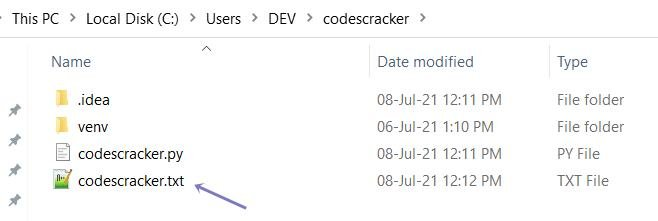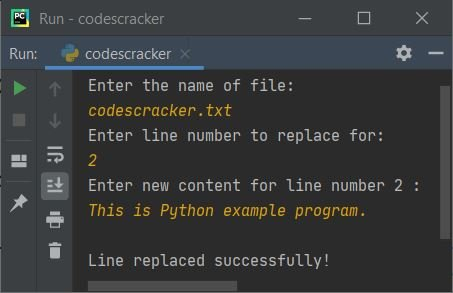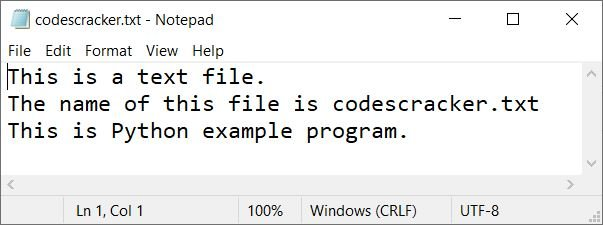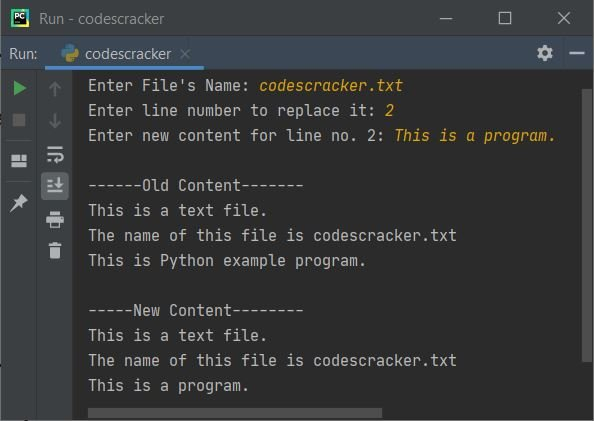# Python Program to Replace Specific Line in File

This article deals with some programs in Python that replaces specific line from a file. Here are the list of programs covered in this article:

• Replace specific line in a file
• Replace specific line in a file and print the new content of file

### Things to do before Program

Since the program given below operates on file, therefore a file must required before executing the program given below. Therefore, create a file name codescracker.txt and put the following content:

```This is a text file.
The name of this file is codescracker.txt
You are practicing Python by example here```

inside that file. Save the file inside the current directory. Here current directory means, the folder where the program's source code is saved. Here is the snapshot of the file stored in current directory:and here is the snapshot of opened file named codescracker.txt:Now let's move on and create the program to operate on this file to replace any line with new line.

Note - To replace any line, you've to enter the line number and then the content to replace that line number with given content.

## Replace Specific Line from File

The question is, write a Python program that replaces any specific line from a file. The name of file, line number, and new content for the line number must be entered by user at run-time. The program given below is answer to this question:

```print("Enter the name of file: ")
filename = input()

filehandle = open(filename, "r")
filehandle.close()

print("Enter line number to replace for: ")
lineNo = int(input())
print("Enter new content for line number", lineNo, ": ")
newline = input()
listOfLines[lineNo] = newline

filehandle = open(filename, "w")
filehandle.writelines(listOfLines)
filehandle.close()

print("\nLine replaced successfully!")```

Note - Use indexing while entering the line number. That is, if you want to replace first line of text file, then enter 0 as input. If you want to replace second line, then enter 1, and so on.

The snapshot given below shows the initial output produced by above Python program. Let's have a look on it:Now provide the inputs, codescracker.txt as name of file, 2 as line number, and This is Python example program. as content to replace with. Here is its sample run with exactly these inputs:Now if you'll see the content of file, codescracker.txt, its third line gets replaced with new line as entered above. Here is the new snapshot of the same file:## Replace any Line in File and Print New Content

In this program, I've implemented the code that prints both the old and new content of file after replacing the line. As it looks more interactive.

```print("Enter File's Name: ", end="")
fname = input()

fhandle = open(fname, "r")
fhandle.close()

print("Enter line number to replace it: ", end="")
lno = int(input())
print("Enter new content for line no.", lno, "\b: ", end="")
nline = input()

print("\n------Old Content-------")
content = ""
content = content.join(lines)
print(content)

lines[lno] = nline
fhandle = open(fname, "w")
fhandle.writelines(lines)
fhandle.close()

print("\n-----New Content--------")
content = ""
content = content.join(lines)
print(content)```

Here is its sample run with user input, codescracker.txt as file's name, 2 as line number, This is a program. as new line to replace with:Python Online Test

« Previous Program Next Program »